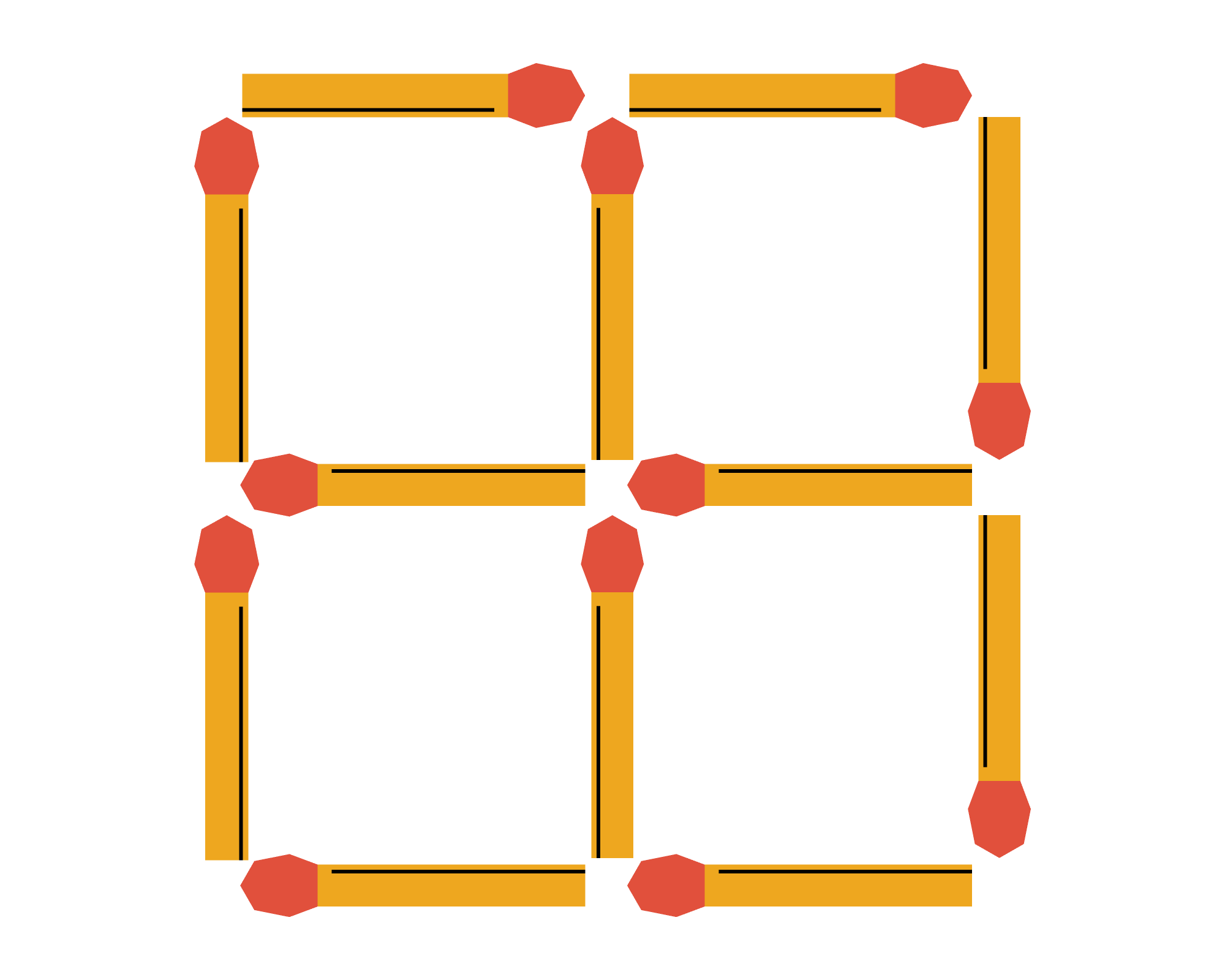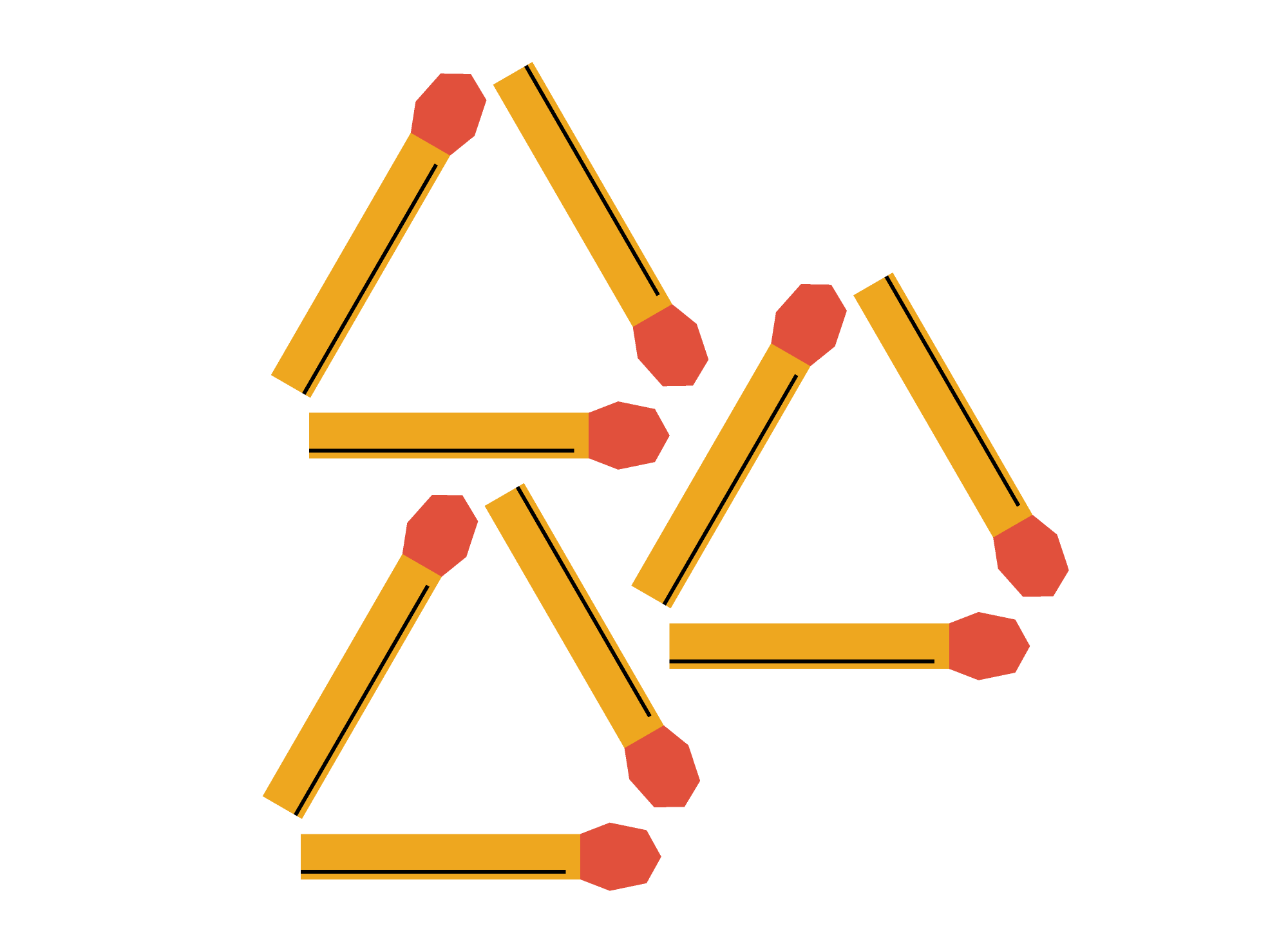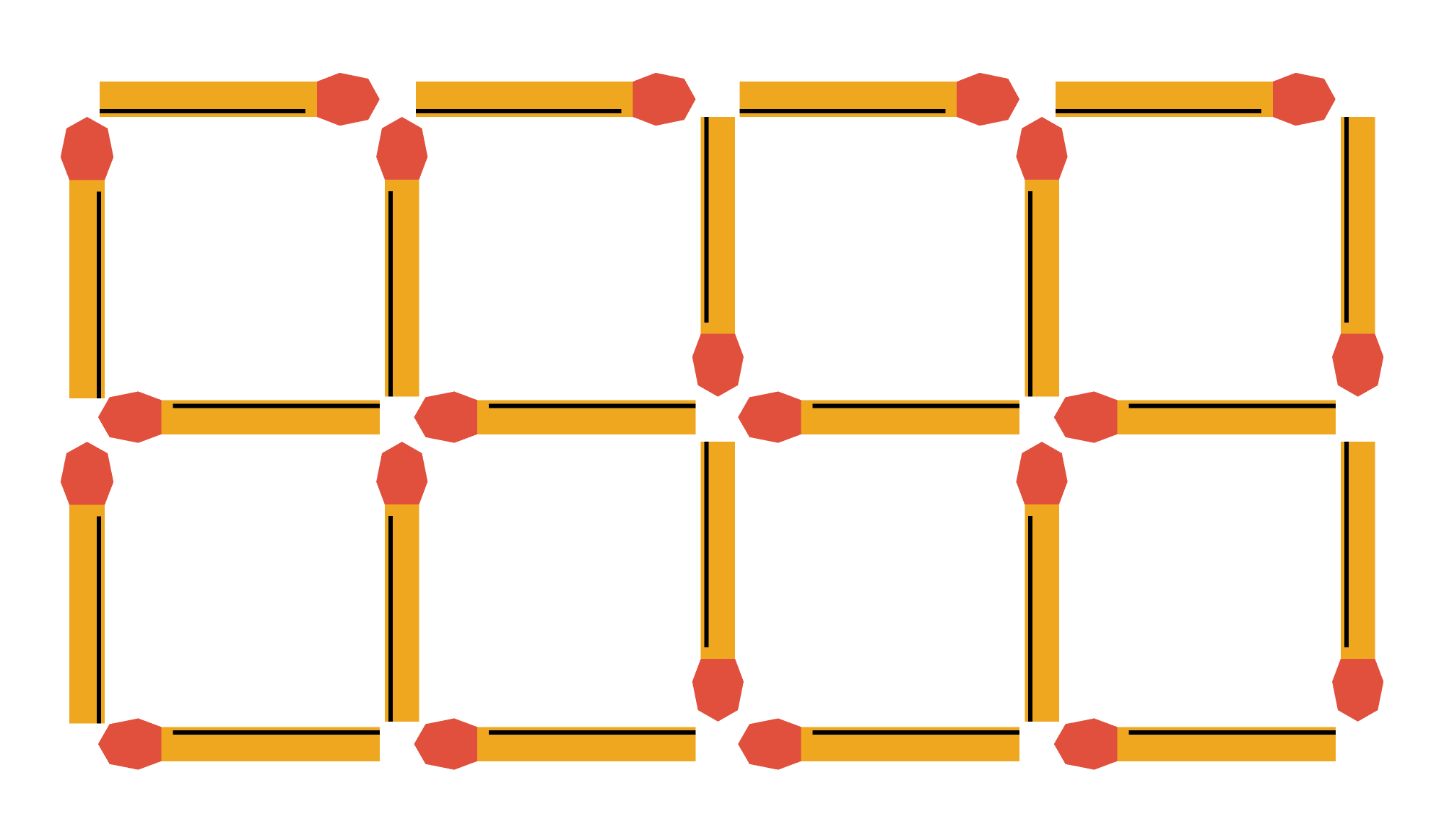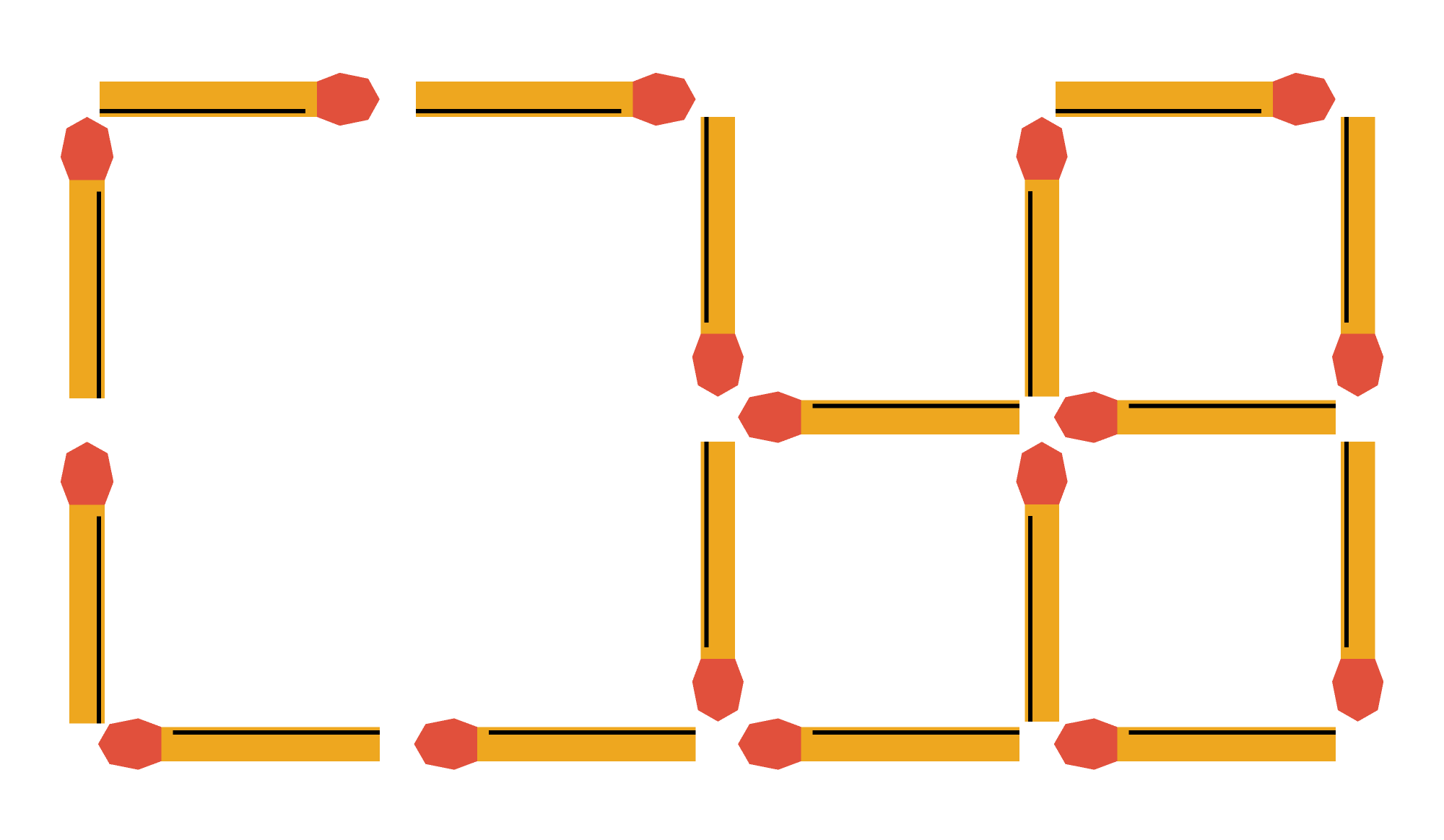## Playing with Matchsticks

It's not obvious at first, but this matchstick figure contains five squares, not four. Can you see them all?When dealing with matchstick puzzles, in addition to thinking about shapes that overlap, we must also think about the possibilities of having shapes of different sizes. For example, this matchstick puzzle does not have any overlapping figures, but it does have a total of four equilateral triangles — three larger triangles and one smaller one:Let's look at one more.

Is it possible to remove $5$ matchsticks to create $4$ squares?Note that there is not any indication in the problem statement that the squares must be the same size. Therefore, we need to consider the possibility that some squares may be different sizes.

Given that we are only removing matchsticks, the possibilities for square sizes include $1 \times 1$ and $2 \times 2.$ By removing four "internal" matchsticks, we create one empty $2 \times 2$ square. Then, by removing one more matchstick from the perimeter, we have three small $1 \times 1$ squares, for a total of four squares.It's easy to get trapped in our own assumptions in these kinds of puzzles, so try to think creatively on the problem below.

# Today's Challenge

What is the minimum number of matchsticks that must be removed from this arrangement to leave exactly two squares?